AP State Board Syllabus AP SSC 10th Class Maths Textbook Solutions Chapter 7 Coordinate Geometry Ex 7.3 Textbook Questions and Answers.

## AP State Syllabus SSC 10th Class Maths Solutions 7th Lesson Coordinate Geometry Exercise 7.3

### 10th Class Maths 7th Lesson Coordinate Geometry Ex 7.3 Textbook Questions and Answers

Question 1.
Find the area of the triangle whose vertices are
i) (2, 3), (-1, 0), (2,-4)
Given: A (2, 3), B (- 1, 0) and C (2, – 4) are the vertices of a △ABC.
Area of the triangle ABC = $$\frac{1}{2}\left|\mathrm{x}_{1}\left(\mathrm{y}_{2}-\mathrm{y}_{3}\right)+\mathrm{x}_{2}\left(\mathrm{y}_{3}-\mathrm{y}_{1}\right)+\mathrm{x}_{3}\left(\mathrm{y}_{1}-{\mathrm{y}}_{2}\right)\right|$$
= $$\frac{1}{2}|2(0+4)-1(-4-3)+2(3-0)|$$
= $$\frac{1}{2}|8+7+6|$$
= $$\frac{21}{2}$$
= 10$$\frac{1}{2}$$ sq.units

ii) (-5, -1), (3, -5), (5, 2)
Given: A (- 5, – 1), B (3, – 5) and C (5, 2) are the vertices of △ABC.
Area of the △ABC
= $$\frac{1}{2}\left|\mathrm{x}_{1}\left(\mathrm{y}_{2}-\mathrm{y}_{3}\right)+\mathrm{x}_{2}\left(\mathrm{y}_{3}-\mathrm{y}_{1}\right)+\mathrm{x}_{3}\left(\mathrm{y}_{1}-{\mathrm{y}}_{2}\right)\right|$$
= $$\frac{1}{2}|-5(-5-2)+3(2+1)+5(-1+5)|$$
= $$\frac{1}{2}|35+9+20|$$
= $$\frac{64}{2}$$
= 32 sq.unitsiii) (0, 0), (3, 0), (0, 2)
Given: O (0, 0), A (3, 0) and B (0, 2) are the vertices of a triangle, △AOB.
Area of the △AOB
= $$\frac{1}{2}\left|\mathrm{x}_{1}\left(\mathrm{y}_{2}-\mathrm{y}_{3}\right)+\mathrm{x}_{2}\left(\mathrm{y}_{3}-\mathrm{y}_{1}\right)+\mathrm{x}_{3}\left(\mathrm{y}_{1}-{\mathrm{y}}_{2}\right)\right|$$
= $$\frac{1}{2}|0(0-2)+3(2-0)+0(0-0)|$$
= $$\frac{1}{2}|6|$$
= 3 sq.units

Or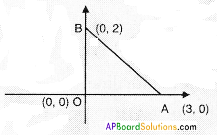△AOB = $$\frac{1}{2}$$ × OA × OB
= $$\frac{1}{2}$$ × 3 × 2
= 3 sq.units

Question 2.
Find the value of ‘K’ for which the points are collinear.
i) (7, -2), (5, 1), (3, K)
Given: A (7, – 2), B (5, 1) and C (3, K) are collinear.
∴ Area of △ABC = 0
But area of triangle
$$\frac{1}{2}\left|\mathrm{x}_{1}\left(\mathrm{y}_{2}-\mathrm{y}_{3}\right)+\mathrm{x}_{2}\left(\mathrm{y}_{3}-\mathrm{y}_{1}\right)+\mathrm{x}_{3}\left(\mathrm{y}_{1}-{\mathrm{y}}_{2}\right)\right|$$
⇒ $$\frac{1}{2} \mid 7(1-\mathrm{K})+5(\mathrm{~K}+2)+3(-2-1)$$ = 0
⇒ $$|7-7 K+5 K+10-9|$$ = 0
⇒ $$|-2 \mathrm{~K}+8|$$ = 0
⇒ -2K + 8 = 0
⇒ -2K = -8
⇒ K = $$\frac{8}{2}$$
i.e., K = 4

ii) (8, 1), (K,-4), (2,-5)
Given: A (8, 1), B(K, – 4) and C (2, – 5) are collinear.
∴ Area of △ABC = 0
⇒ $$\frac{1}{2}\left|\mathrm{x}_{1}\left(\mathrm{y}_{2}-\mathrm{y}_{3}\right)+\mathrm{x}_{2}\left(\mathrm{y}_{3}-\mathrm{y}_{1}\right)+\mathrm{x}_{3}\left(\mathrm{y}_{1}-{\mathrm{y}}_{2}\right)\right|$$ = 0
⇒ $$\frac{1}{2}|8(-4+5)+\mathrm{K}(-5-1)+2(1+4)|$$ = 0
⇒ $$|8-6 \mathrm{~K}+10|$$ = 0
⇒ $$|18-6 \mathrm{~K}|$$ = 0
⇒ 18 – 6K = 0
⇒ 6K = 18
⇒ K = $$\frac{18}{6}$$
i.e., K = 3

iii) (K,K), (2, 3), and (4,-1)
A (K, K), B (2, 3) and C (4, – 1) are collinear.
∴ Area of △ABC = 0
⇒ $$\frac{1}{2}\left|\mathrm{x}_{1}\left(\mathrm{y}_{2}-\mathrm{y}_{3}\right)+\mathrm{x}_{2}\left(\mathrm{y}_{3}-\mathrm{y}_{1}\right)+\mathrm{x}_{3}\left(\mathrm{y}_{1}-{\mathrm{y}}_{2}\right)\right|$$ = 0
⇒ $$\frac{1}{2}|\mathrm{~K}(3+1)+2(-1-\mathrm{K})+4(\mathrm{~K}-3)|$$ = 0
⇒ $$|4K-2-2K+4K-12|$$ = 0
⇒ $$|6 \mathrm{~K}-14|$$ = 0
⇒ 6K – 14 = 0
⇒ 6K = 14
⇒ K = $$\frac{14}{6}$$ = $$\frac{7}{3}$$
∴ K = $$\frac{7}{3}$$Question 3.
Find the area of the triangle formed by joining the mid-points of the sides of the triangle whose vertices are (0, -1), (2, 1) and (0, 3). Find the ratio of this area to the area of the given triangle.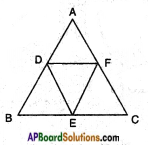Given: A (0, – 1), B (2, 1) and C (0, 3) are the vertices of △ABC.
Let D, E and F be the midpoints of the sides $$\overline{\mathrm{AB}}$$, $$\overline{\mathrm{BC}}$$ and $$\overline{\mathrm{AC}}$$.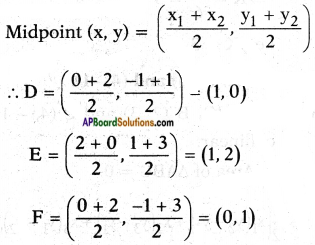Area of a triangle ABC =
$$\frac{1}{2}\left|\mathrm{x}_{1}\left(\mathrm{y}_{2}-\mathrm{y}_{3}\right)+\mathrm{x}_{2}\left(\mathrm{y}_{3}-\mathrm{y}_{1}\right)+\mathrm{x}_{3}\left(\mathrm{y}_{1}-{\mathrm{y}}_{2}\right)\right|$$
= $$\frac{1}{2}|0(1-3)+2(3+1)+0(-1-1)|$$
= $$\frac{1}{2}|8|$$
= 4 sq.units
Area of △DEF = $$\frac{1}{2}|1(2-1)+1(1-0)+0(0-2)|$$
= $$\frac{1}{2}|1+1|$$
= $$\frac{2}{2}$$
= 1 sq.units
Ratio of areas = △ABC : △DEF = 4 : 1.
△ADF ≅ △BED ≅ △DEF ≅ △CEF
∴ △ABC : △DEF = 4 : 1

Question 4.
Find the area of the quadrilateral whose vertices taken inorder are (-4, -2), (-3, -5),(3, -2) and (2, 3).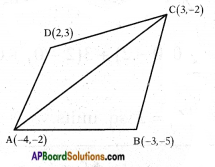Given: A (- 4, – 2), B (- 3, – 5), C (3, – 2) and D (2, 3) are the vertices of the quadrilateral ▱ ABCD.
Area of ▱ ABCD = △ABC + △ACD.
Area of a triangle =
$$\frac{1}{2}\left|\mathrm{x}_{1}\left(\mathrm{y}_{2}-\mathrm{y}_{3}\right)+\mathrm{x}_{2}\left(\mathrm{y}_{3}-\mathrm{y}_{1}\right)+\mathrm{x}_{3}\left(\mathrm{y}_{1}-{\mathrm{y}}_{2}\right)\right|$$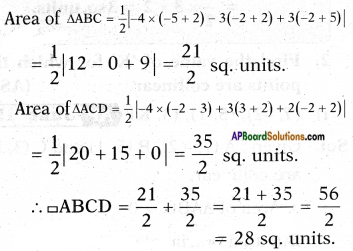Question 5.
Find the area of the triangle formed by the points by using Heron’s formula.
i) (1, 1), (1, 4) and (5, 1)
ii) (2, 3), (-1,3) and (2, -1)
i) (1, 1) (1, 4) and (5, 1)
let A (1, 1) B(l, 4) and C(5, 1) are the vertices then length of sides can be calculated using the formula
$$\sqrt{\left(x_{2}-x_{1}\right)^{2}+\left(y_{2}-y_{1}\right)^{2}}$$
now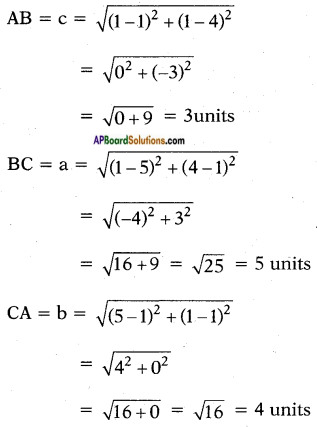now formula for area of triangle using Heron’s formula = △ = $$\sqrt{s(s-a)(s-b)(s-c)}$$
where s = $$\frac{a+b+c}{2}$$
∴ s = $$\frac{3+4+5}{2}$$ = $$\frac{12}{2}$$ = 6
∴ △ = $$\sqrt{6(6-5)(6-4)(6-3)}$$
= $$\sqrt{6 \times 1 \times 2 \times 3}$$
= $$\sqrt{6 \times 6}$$
= 6 sq. units
∴ area of given triangle = 6 sq units

ii) let the vertices of given triangle A (2, 3), B (-l, 3) and C (2, -1)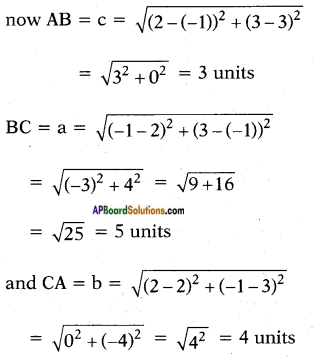∴ a = 5, b = 4, c = 3 units
now from using Heron’s formula area of triangle
= △ = $$\sqrt{s(s-a)(s-b)(s-c)}$$
where s = $$\frac{a+b+c}{2}$$
= $$\frac{5+4+3}{2}$$
= $$\frac{12}{2}$$ = 6
∴ △ = $$\sqrt{6(6-5)(6-4)(6-3)}$$
= $$\sqrt{6 \times 1 \times 2 \times 3}$$
= $$\sqrt{6 \times 6}$$
= 6 sq. units
∴ area of given triangle = 6 sq units